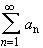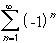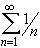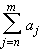#Interactive Real Analysis

Next | Previous | Glossary | Map

## 4.2. Convergence Tests

### Divergence Test

If the seriesconverges, then the sequenceconverges to zero. Equivalently:

If the sequencedoes not converge to zero, then the seriescan not converge.

This test can never be used to show that a series converges. It can only be used to show that a series diverges. Hence, the second version of this theorem is the more important, applicable statement.

 Examples 4.2.2:Does the Divergence test apply to show that the seriesconverges or diverges ? How about convergence or divergence of the series?
Proof:

Suppose the series does converge. Then it must satisfy the Cauchy criterion. In other words, given any> 0 there exists a positive integer N such that whenever n > m > N then

|| <Let m > N and set n = m. Then the series above reduces to
| a n | <if n > N. That, however, is saying that the sequenceconverges to zero.Next | Previous | Glossary | Map